﻿ Modeling of Ionization Energy of Elements Using Hartree-Fock<i> </i>Method: An Introduction to Computational Quantum Chemistry for Undergraduate StudentsPublications are Open
Access in this journal
Article Versions
Export Article
• Normal Style
• MLA Style
• APA Style
• Chicago Style
Research Article
Open Access Peer-reviewed

### Modeling of Ionization Energy of Elements Using Hartree-FockMethod: An Introduction to Computational Quantum Chemistry for Undergraduate Students

Krishnamohan G P , Thomas Mathew, Simi Saju, James T. Joseph
World Journal of Chemical Education. 2017, 5(3), 112-119. DOI: 10.12691/wjce-5-3-6
Published online: May 26, 2017

### Abstract

The periodic properties of elements are central to the concept of chemistry and are deeply depending on the electron configuration of atoms. Among the common periodic properties such as atomic radii, electronegativity, electron affinity, the ionization energy of elements gives a clear trend in periodicity. Here, we present a classroom activity, mainly for undergraduate students, to simulate first ionization energy as an important periodic property of elements (up to xenon atom). A set of Hartree-Fock calculations were performed with Gaussian 03 suit of programs and the results have been compared with that of the experimental values. While comparing the experimental and calculated values, we have stressed the role of central-field approximation in the HF theory, important features of Slater type orbitals near the nucleus and the significance of electron correlation in multi-electron systems. Some suggestions for improvement of the results are also discussed.

### 1. Introduction

The modern periodic table is one of the most perfectly arranged data at our scientific disposal and a fundamental source of information for a chemist 1, 2. For centuries, chemists had been working to shed light on the systematic arrangement of the elements 3. The similarities in numerous physical and chemical properties of elements led to the concept of periodicity of elements. However, the arrangement of elements in the modern periodic table is solely based on the electron configuration of atoms. Here, as a first time computational chemistry laboratory activity to undergraduate students, we simulated the periodicity of first ionization energy (IE) of elements with Gaussian electronic structure program 4. The calculated ionization energies were compared against experimental values and found a semi-quantitaive agreement between the theory and the experiment.

The motivation to write this article is the following. In general, ab initio computational chemistry is introduced to under-graduate curriculum (chemistry major) by introducing Hartre-Fock (HF) method and, nowadays, often accompanied with density functional theory. As an immediate application to these methods, most of the text books 5, 6, 7 treat molecular systems and calculates the properties such as equilibrium structure, molecular energy levels, shapes of molecular orbitals etc. However to fully understand the results from these calculations, the students are expected to have a good knowledge in molecular orbital (MO) theory along with the group theory (for instance, to analyze molecular orbitals, or bonding). In fact, almost every molecular quantum chemistry package is heavily depending on the symmetry-adapted subroutines since it substantially speed-up the ab initio calculations. But in this computational work, students with no knowledge of these theories can participate as well and learn the basic terms or concepts of computational chemistry (such as the idea of a basis set, difference between Slater type orbitals (STO) and Gaussian type orbitals (GTO), significance of electron correlation etc). Since these single-atom calculations take only a short time (typically less than two minutes for each atom on a standard 2 GHz PC), every student in the class can do this computational work independently. Though the students are not expected to have a prior knowledge in Gaussian program, we strongly recommend that the teacher/demonstrator should teach them some basic key-words as well as the structure of the Gaussian input/output file before the calculations (See the supplementary information for a sample atomic UHF calculation input file for Mg atom).

### 2. Computational Method

The first IE of an atom is defined as the minimal energy required to remove the outermost valence electron from its isolated gaseous atom. To simulate IE, we used spin-unrestricted HF 7 method in conjunction with the Koopmans theorem. Note that one can also use other ab initio codes such as GAMESS, HyperChem, QChem for doing this computational project. According to Koopman’s theorem 8 the ionization energy of atom, I, is approximately equal to the negative value of its highest occupied atomic energy level (ϵ), viz.,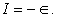And generally Koopmans theorem gives good qualitative trend for IE but not for electron affinity energies. One major advantage of the Koopmans approach is that only computation of the neutral species is required to calculate IE. Although the theorem is originally proposed for the closed shell systems, later it was also formulated for the open systems 9. It is also interesting to note that there are two (almost-) equal and opposite factors (electron correlation energy, EC (see Appendix A) and the energy from the orbital relaxation) are operating in the process of calculating IE from the Koopmans theorem. Refer 10 for more details.

The HF is a method to solve many-body (Schrodinger) equation and gives approximate wavefunctions for many electron/fermionic systems. Historically, Hartree and Hartree-Fock calculations had been extensively and exclusively used to analyze atomic spectra 11. We would like to stress that the HF formalism of Gaussian as well as other popular molecular electronic structure codes is different from the original form of HF as it is developed by Hartree and Fock for atomic systems. In the modern electronic structure program like Gaussian, a matrix form of the Hamiltonian is used (because of the usage of finite basis set) but in the original formalism of Hartree and Fock, they solved the atomic radial equation (as an integro-differential equation) by using numerical quadrature, and using analytical Slater type orbitals 12.

However, in both formalisms, the variational principle is used to get improved eigenvalues (i.e. the atomic energy levels) along with two approximations, viz, (1) the Born-Oppenheimer approximation and (2) the Central-field approximation 13. As the description of the Born-Oppenheimer approximation can be seen in any standard text book on the quantum chemistry, we only shortly describe it here. The total Hamiltonian of an atom (or a molecule) can be written with usual notations as,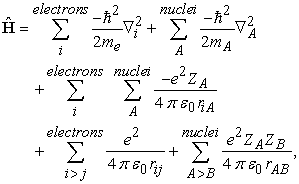(1)

where the terms are representing the total kinetic energy of the electrons, total kinetic energy of the nuclei, electrostatic interaction between the electrons and the nuclei, electrostatic interaction between the electrons and electrostatic interaction between the nuclei, respectively. Since the mass of nucleus is very high compared (approximately 2000 times higher) to electrons we can imagine that the nucleus of the atom(s) is practically not moving. This will remove the second term (total kinetic energy of the nuclei) and hence simplify the Hamiltonian as shown below,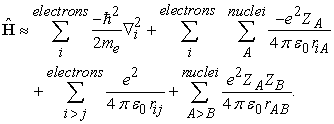(2)

For example, if we apply the BO approximation to helium atom, the Hamiltonian of the He atom will have only six Cartesian degrees of freedom and not the nine degrees of freedom (six from its two electrons and three from the nucleus) thus the approximation will clearly simplify the Hamiltonian and corresponding eigenfunctions.

The central field approximation is a major approximation in HF theory. Note that because of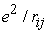term in the Hamiltonian we cannot separate the Hamiltonian to obtain N (where N is the total number of electrons in an atom) independent set of equations in the coordinates of each and every electron. One way to resolve this issue is to apply the central field approximation. In this approximation, an electron is considered moving independently of the other electrons in an average potential or effective field which is created by other electrons and the nucleus. This average potential can be written for an atomic system as 13,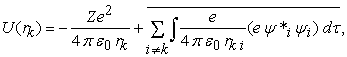(3)

where, Z is the atomic number of the element, rk is the position of k’th electron, and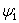is the normalized total wavefunction of the i’th electron (which contains both the radial and angular parts). Because of this approximation, for an N electron atomic system, the total Hamiltonian can be written as the sum of N independent particle Hamiltonian as,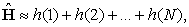where, h(k), being an independent particle Hamiltonian, is defined as,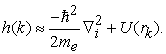(4)

Note that the mathematical form of central field, (as shown in Equation (3)) – which is independent of angles so the shape of U(rk) is spherically symmetric 5, 6. See Figure 1 for schematic diagram of U(rk) of lithium atom. Because of this approximation, the force exerted by e3 (the third electron) on e1 and e2 are same. In other words the ‘instantaneous’ interaction ((Coulomb-) electron correlation) between the electrons are not treated in the HF method. This absence of electron correlation is a fundamental source of error in the HF method (see Appendix A).

• Figure 1. A ‘snap shot’ of the lithium atom. The spherical color gradient represents the central field, U(rk). The structure of the central field may vary with the atomic number

A major problem that we faced in this project is the choice of suitable basis set. In Gaussian program one can only use GTOs (Gaussian type orbitals) and not STOs (Slater type orbitals). See Appendix B for a comparison of these basis sets. Moreover, only few basis sets (3-21G, DGDZVP and UGBS) are available to span a sizable range (elements up to xenon) of atoms 4 to construct the familiar IP trend in a consistent manner. And by considering the simplicity of 3-21G basis set we used this set for our calculations. For preparing a series of G03 input files, a platform independent JAVA program has been written. This program, GJFMake (available from the authors) is used to extract some important data such as total energy, highest occupied atomic orbital energy etc. (see supplementary information).

### 3. Results and Discussions

The IE from the UHF/3-21G calculations along with the experimental values 14 is shown in the Figure 2. The graph shows a semi-quantitative agreement between HF theory and the experiment (See Table 1). Since we can interpret ionization energies as a proof for the existence of electronic shells and sub-shells of atoms one can also imply that these shells and sub-shells are also retained in the HF theory.

• Figure 2. The experimental and calculated IE value of atoms (H-Xe)

For noble gas elements (He, Ne, Ar, Kr, Xe), the experimental values of IE are very well matched with that of HF values. Similarly, the IE values of alkali metals (Li, Na, K, Rb) also agree with the experimental values. It is also clear from the figure that the elements with fully half-filled (sub-shell-) electron configuration give good IE values (for example, N, P, As, Sb).

The IE of 3d elements (Sc-Zn), and, particularly 4d elements (Y-Cd) are not well reproduced by the HF method. This feature can be due to the following factors: (1) The larger correlation energy (note that Ec increases with the number of electrons and Ec indicates the deviation of HF energy from the possible theoretical minimum). And it indicates that more accurate theories are needed which include electron correlation (such as Moeller-Plesset perturbation methods or other post-HF theories), (2) insufficient basis set. Note that 3-21G basis set is better than STO-nG set, to get more accurate results we have to use more accurate sets such as 6-311G. However higher basis set (e.g. 6-311G) are not available for all the elements in G03 program, and (3) limitations of Koopman’s theorem (see the last part of this section on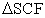procedure).

The deviation of IE values of 3d and 4d elements can be justified in terms Unsold theorem 6, which states,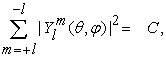where,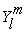is the spherical harmonics function and C is a positive constant (since C is independent of angles, the shape of this summation will results a sphere). For an illustrative purpose, the Unsold theorem can be depicted for 3d spherical harmonics as shown in Figure 3.

• Figure 3. An illustration of Unsold theorem of normalized 3d spherical harmonics functions

Moreover, analytically, we can easily verify that,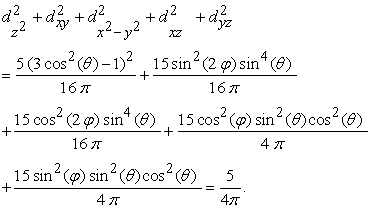It can be seen that the second term of U(rk) is nothing but the average electron-electron repulsion which can be represented by spherically-symmetrical concentric shells. These concentric shells are thus comprised of various shells and sub-shells in an atom. Also note that in HF theory one can calculate electron densities (of various shells such as K, L, M, …) and it agrees well with the experimental electron densities obtained from the electron diffraction experiment 15.

Before proceeding further, there are three points that we would like to stress, viz,

1. We employed UHF (one-determinant UHF theory as it is implemented in the Gaussian) method, and in this case the U(rk) is perfectly spherically symmetric if the atomic shells are either completely filled or half-filled.

2. A symmetry-breaking will occur if the atom has an incompletely filled shell 16. This will immediately follow that the resultant solutions of this UHF Hamiltonian is no longer have the pure s, p, d symmetry properties of a completely spherical U(rk). (one way to avoid this is to use a multi-determinant wavefunction. Any further discussions on this, however, are beyond the scope of this paper)

3. Because of the above point the shape of electron density of these atoms (with incomplete shells) are not spherical (since Unsold theorem hold only for pure spherical harmonics). It is also interesting to note that these types of atoms may have a non-zero angular momentum so that its Hamiltonian is not-invarient (i.e. not-spherical) under rotations 17.

Using these facts, we can see that, in the case of noble gases (and other elements with completely half-filled shells), the second term of U(rk) corresponds to perfectly concentric spherical shell and, conversely, these concentric shells will have some degree of corrugation (i.e. it will be symmetric but not perfectly spherical) in the case of other electron configurations. For example, this effect (i.e. deviation from the perfectly symmetric shell) will be enhanced for 3d elements and it will be even more intensified in the case of 4d elements (4d orbital shape is much more diffused than 3d orbital). Note that in HF theory, atomic structure is described in terms of orbitals based on a spherically symmetric effective potential U(rk) - which ideally happens only for inert gas atoms. This will partially account for, (1) the surprisingly good agreement between theoretical and calculated IE values of noble gas elements and (2) average deviation of IE values of 3d or 4d elements.

In this paper we have discussed the ionization energy of atoms calculated by spin-unrestricted HF methods. These results can be systematically improved by considering electron correlation and thus using post-HF methods such as Møller–Plesset perturbation theory or multi-reference methods. In addition to this, a more accurate approach to calculate IE, such asprocedure 18, can be employed to get better results, where IE can be evaluated as,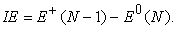Here, E+(N-1) and E0(N) correspond to the total energies of ionized and neutral atoms, respectively. For example, the experimental IE of beryllium atom is 9.32 eV and this value cannot be calculated by using Koopmans theorem, which is partially due to the neglect of orbital relaxation. And if we use acalculation using a configuration interaction method with a correlation-consistent basis sets (e.g. CISD/cc-pVTZ) one can get a very accurate IE value (9.28 eV). Thus the reader is advised to do this calculation for the 3d, as well as 4d elements and for O, Mg, S and Ca atoms.

### 4. Conclusion

A qualitative introduction to HF theory for atomic systems and its application in modeling of atomic (first-) ionization energies are discussed in this paper. Experimental and simulated values were found to agree semi-quantitatively. The role of central-field approximation in HF theory to interpret the IE values of noble gas elements have been discussed in terms of Unsold theorem. The possible cause of deviation of IE values of 3d and 4d elements are noted. Some possible improvements to get more accurate simulated IE values are also mentioned.

### Competing Interests

The authors declare no competing financial interest.

### Appendices

[A] Electron correlation

Since the HF method (doesn’t matter whether it is a UHF, RHF or ROHF) neglects instantaneous position of electrons, an error will always be present in HF theory. Lowdin quantified this error by defining correlation energy 19 as,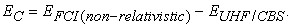Note that (a) EFCI is not an experimentally determined quantity and in practice FCI (the full configuration interaction) calculation is not possible except for very small molecules or atoms (in other words, truncated-CI is often used for calculating EC for molecular systems. EUHF/CBS is the energy obtained by using a complete basis set in a spin un-restricted HF calculation) and (b) EC becomes larger for the systems with the larger number of electrons and finally, (c) it will always be a negative quantity.

An illustration of EC (of helium atom) is depicted in the Figure 4. Here, EFCI(non-relativistic) is almost equal to the experimental binding energy of He atom 6. It can be seen from our calculation that the value of EC is -0.0434 Hartree (or -1.18 eV) and the HF method has an error of 1.45%. But this is still an approximate value (since 6-311++G** basis set is not a complete basis set), and this value can be still lowered if one use a Slater function instead of a Gaussian basis. For example, the lowest reported value of EC of helium is -0.0420 Hartree (-1.14 eV) 20, 21 because of the double-zeta Slater basis set in the calculation.

• Figure 4. The plot shows the total energy (in Hartree) of helium atom and the methods used to calculate the values (from left to right, each point corresponds to UHF/STO-3G, UHF/STO-6G, UHF/4-31G**, UHF/6-311++G**, UMP2/6-311G**, UMP4/6-311++G**, UQCISD(TQ)/6-311++G** and Full CI (equivalent to experimental)). The yellow line is a trend line
[B] Evaluation of Kato’s cusp condition for Hydrogen atom: Difference between STO and GTO orbitals

In this section we shall prove that (a) the Katos cusp condition (see below) will be satisfied if one use a complete solution of Hamiltonian (e.g. a Slater orbitals which contains both angular and radial part), and (b), Gaussian orbitals (such as a STO-3G basis) will not satisfy cusp equation.

In atomic and molecular physics, a coalescence point is defined as the point where Coulomb singularity can occur 22. For example, if the position of 1s electron approaches to zero with respect to nucleus, the potential energy term will tend to negative infinity (i.e. the nucleus-electron potential term become diverge). To compensate this effect the kinetic energy of 1s electron will also diverge (to positive infinity) at this region so that the sum of kinetic and potential energies is finite (as it should be for any stable system). For the 1s electron of hydrogen atom, we can prove that (by using spherical coordinates):

If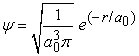, then,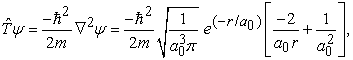and, at the center of the atom the above value becomes,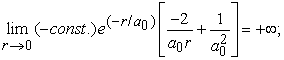which demonstrates that the kinetic energy diverges to plus infinity, if the position of electron (r) tends to zero with respect to the nucleus. Note that at this point, the curvature of the wavefunction, which is approximately proportional to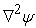, is +∞ and it clearly indicates that the wavefunction must have a discontinuity in slope (also known as cusp) at the origin 5.

Furthermore, these situations can be easily generalized (as a mathematical constraint), and is usually known as Katos (nucleus-electron) cusp condition. This cusp condition is usually written in terms of the electron density (n(r)) as shown below (for detailed derivation see, 23),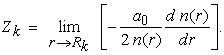Here, Zk is the atomic number of the nucleus or the total positive charge of the nucleus, r is the position of the electron and the Rk is the position of the k’th nucleus.

In the case of the hydrogen atom, the normalized total wavefunction of 1s orbital is defined as,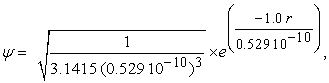and the derivative of the electron density is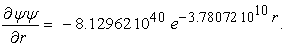Finally the limit can be evaluated as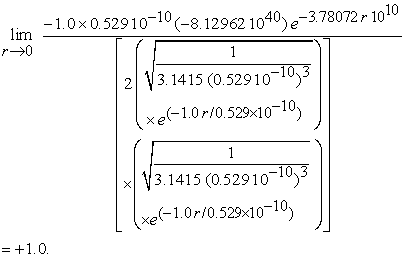Note that to evaluate the limit we didn’t use atomic unit.

The following function is the normalized STO-3G basis set (in SI unit, where a0=0.529 10-10m) for the hydrogen atom (See 10 for the numerical values for the coefficients and the exponents):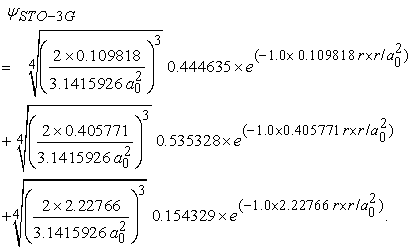It can be easily proved that,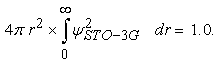Since,∂/∂r (e(-constant.r.r)) = 0, the Katos cusp condition is not valid for STO-3G basis set, in other words,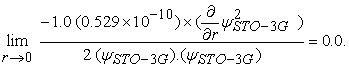This can be further generalized for other Gaussian basis sets such as 3-21G, 6-311G etc. And this is left to the reader as an exercise.

Thus, Katos condition can be regarded as a method to determine the quality of the basis set. Note that, for brevity, we only discussed nucleus-electron cusp condition and for the details of electron-electron cusp condition the reader is advised to refer 23.

### References

  Wang, S., and Schwarz, W. H. E., “Icon of Chemistry: The Periodic System of Chemical Elements in the New Century,” Angew. Chem. Int. Ed., 48, 2, 2009. In article View Article  Schwarz, W. H. E., and Rich, R. L., “Theoretical Basis and Correct Explanation of the Periodic System: Review and Update,” J. Chem. Educ., 87, 435, 2010. In article View Article  Scerri, E. R., The Periodic Table: Its Story and Its Significance, Oxford University Press, 2007. In article View Article  Frisch, M. J. et al., Gaussian 03, revision E. 01, Gaussian Inc., Wallingford CT, 2004. In article  Atkins, P., and de Paula, J., Physical chemistry, 7/e. W. H. Freeman, 2001. In article  McQuarrie, D. A., Quantum chemistry, 2/e. University Science Books, 2008. In article  Levine, I. N., Quantum chemistry, 5/e. Prentice-Hall of India, 1999. In article  Koopmans, T., “On the assignment of wave functions and eigenvalues to the individual electrons of an atom”, Physica, 1, 104, 1934. In article View Article  Plakhutin, B. N., Gorelik, E. V., and Breslavskaya, N. N., “Koopmans' theorem in the ROHF method: Canonical form for the Hartree-Fock Hamiltonian,” J. Chem. Phys., 125 (20), 204110, 2006. In article View Article  PubMed  Szabo, A., and Ostlund, N. S., Modern Quantum Chemistry: Introduction to Advanced Electronic Structure Theory, McGraw-Hill, 1989. In article View Article  Condon, E. U., and Shortley, G. H., The Theory of Atomic spectra, Cambridge University Press, 1964. In article PubMed  Fischer, C. F., Brage, T., and Johnsson, P., Computational Atomic Structure: An MCHF Approach, CRC Press, 1997. In article View Article  Woodgate, G. K., Elementary Atomic Structure, Oxford University Press, 1983. In article  http://physics.nist.gov/PhysRefData/ASD/ionEnergy.html for the experimental ionization data of elements and its references (accessed on 23/04/2017). In article View Article  Bartell, L. S., and Brockway, L. O., “The Investigation of Electron Distribution in Atoms by Electron Diffraction,” Phys. Rev., 90, 833, 1953. In article View Article  McWeeny, R., and Sutcliffe, B. T., “Fundamentals of Self-Consistent-Field (SCF), Hartree-Fock (HF), Multi-Configuration (MC) SCF and Configuration Interaction (CI) schemes,” 1985, Comput. Phys. Report., 2 (5), 217, 1985. In article View Article  Solovej, J. S., “The size of atoms in Hartree-Fock theory” in Partial Differential Equations and Mathematical Physics: The Danish-Swedish Analysis Seminar, Springer, 1995, 321. In article View Article  Jones, R. O., and Gunnarsson, O., “The density functional formalism, its applications and prospects,” Rev. Mod. Phys., 61, 689, 1989. In article View Article  Popelier, P., Solving the Schrödinger Equation: Has Everything Been Tried? Imperial College Press, 2011. In article View Article  Clementi, E., and Raimondi, D. L., “Atomic Screening Constants from SCF Functions,” J. Chem. Phys., 38, 2686, 1963. In article View Article  Clementi, E., “Simple basis set for molecular wave functions containing first- and second-row atoms,” J. Chem. Phys., 40, 1944, 1964. In article View Article  Reinhardt, P., and Hoggan, P. E., “Cusps and derivatives for wave-functions expanded in Slater orbitals: A density study,” Int. J. Quantum. Chem., 109, 3191, 2009. In article View Article  Helgaker, T., Jorgensen, P., and Olsen, J., Molecular Electronic-Structure Theory, Wiley, 2000. In article View Article

### Supplementary Informations

The above Table shows a sample input for the Gaussian calculation of Mg (neutral) atom. Note that the line numbers are not the part of the Gaussian input and the last blank line is a mandate for some versions of Gaussian program. The first line indicates the method and basis set and the 5th and 6th lines indicate the charge and spin-multiplicity of the atom, and the Cartesian position of the atom, respectively.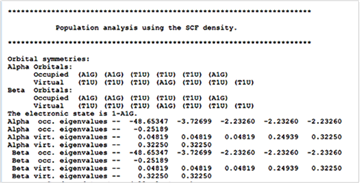The energy of the highest occupied level of an atom can be extracted from the output file from the G03 program, which is usually printed below the banner, “Population analysis using SCF density”. In the case of Mg atom, it is -0.25189 Hartee (all the negative values indicate that the electrons with these energies are in the occupied levels) and note that there are 12 negative numbers. In this case, according to Koopman’s theorem, IE is, -1 × (-0.25189) × 27.211 = + 6.85 eV.This work is licensed under a Creative Commons Attribution 4.0 International License. To view a copy of this license, visit http://creativecommons.org/licenses/by/4.0/